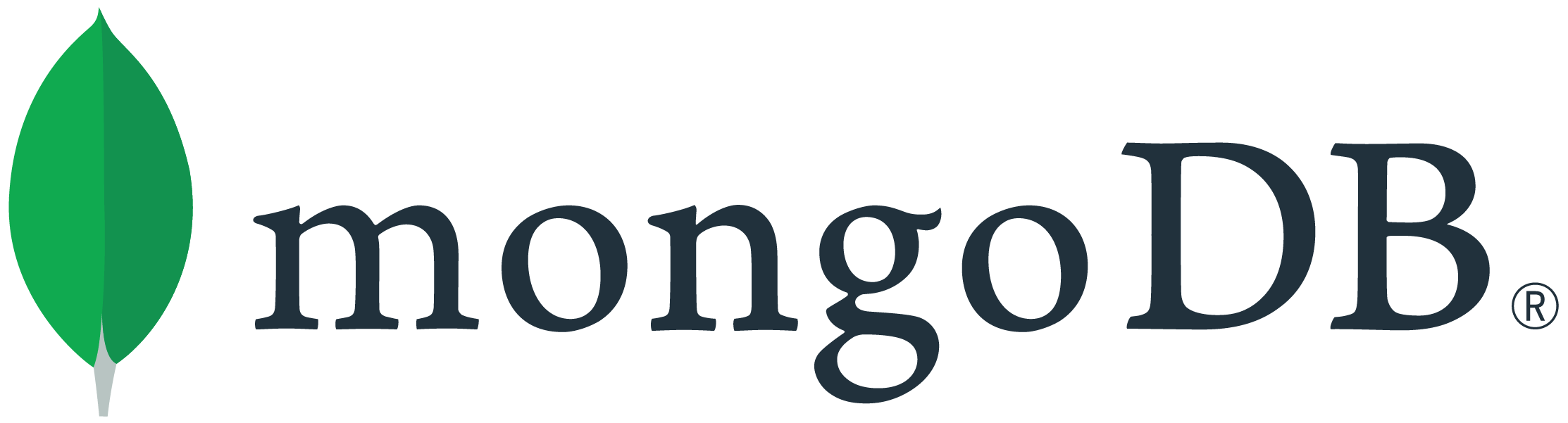# Chapter 4: Quiz 2: \$expr. Wrong solution?

Shouldn’t the second answer be wrong?

\$lt is not the opposite of \$gt as the solution states:

\$let would be correct, right? However as statet \$lt should be wrong…

1 Like

I think the quiz is correct.

a > b is equivalent to b < a while a >= b is equivalent to b <= a

Which translate to

{ \$gt : [ a , b ] } being equivalent to { \$lt : [ b , a ] }
while { \$gte : [ a , b ] } being equivalent to { \$lte : [ b , a ] }

2 Likes

Yes, I think you are rightThank you!